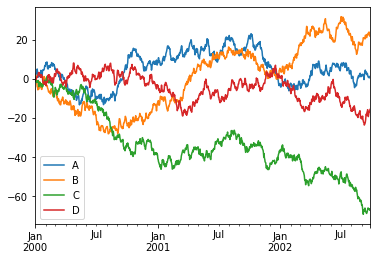# Introduction¶

The PyData ecosystem has a number of core Python data containers that allow users to work with a wide array of datatypes, including:

• Pandas: DataFrame, Series (columnar/tabular data)
• XArray: Dataset, DataArray (multidimensional arrays)
• Dask: DataFrame, Series, Array (distributed/out of core arrays and columnar data)
• Streamz: DataFrame(s), Series(s) (streaming columnar data)
• Intake: DataSource (remote data)

Many of these libraries have the concept of a high-level plotting API that lets a user generate common plot types very easily. The native plotting APIs are generally built on Matplotlib, which provides a solid foundation, but means that users miss out the benefits of modern, interactive plotting libraries for the web like Bokeh and HoloViews.

hvPlot provides a high-level plotting API built on HoloViews and Bokeh that provides a general and consistent API for plotting data in all the abovementioned formats.

As a first simple illustration of using hvPlot, let's create a small set of random data in Pandas to explore:

In :
import numpy as np
import pandas as pd

index = pd.date_range('1/1/2000', periods=1000)
df = pd.DataFrame(np.random.randn(1000, 4), index=index, columns=list('ABCD')).cumsum()


Out:
A B C D
2000-01-01 -0.317196 1.740124 0.563833 -0.563049
2000-01-02 0.934648 1.794659 -0.507286 -0.474963
2000-01-03 1.437368 1.852434 -1.100336 -0.469829
2000-01-04 2.698701 -0.100714 -1.022462 -0.082626
2000-01-05 2.831587 -1.178018 -1.837544 0.373910

## Pandas default .plot()¶

Pandas provides Matplotlib-based plotting by default, using the .plot() method:

In :
%matplotlib inline

df.plot();The result is a PNG image that displays easily, but is otherwise static.

NOTE: In recent versions of pandas (later than 0.25.0), the backend can be swapped out using pd.options.backend.plotting == 'holoviews', to learn more about this see Pandas API.

## .hvplot()¶

If we instead change %matplotlib inline to import hvplot.pandas and use the df.hvplot method, it will now display an interactively explorable Bokeh plot with panning, zooming, hovering, and clickable/selectable legends:

In :
import hvplot.pandas  # noqa

df.hvplot()